# Mass-spring system: cardinality function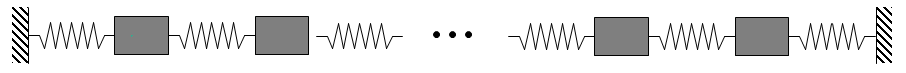Mass-spring system with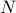masses on a line.

Here, we show the Matlab code and the computational results obtained using lqrsp.m. In this example, we use the cardinality function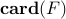to promote sparsity. We set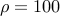and select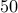logarithmically-spaced points for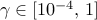.

## Matlab code

```% State-space representation of the mass-spring system with N = 50 masses
N = 50;
I = eye(N,N);
Z = zeros(N,N);
T = toeplitz([2 -1 zeros(1,N-2)]);
A = [Z I; -T Z];
B1 = [Z; I];
B2 = [Z; I];
Q = eye(2*N);
R = 10*I;

% Compute the optimal sparse feedback gains
options = struct('method','card','gamval',logspace(-4,0,50),...
'rho',100,'maxiter',100,'blksize',[1 1]);
tic
solpath = lqrsp(A,B1,B2,Q,R,options);
toc
```

## Computational results

### Sparsity patterns

The number of nonzero elements in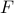decreases with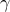.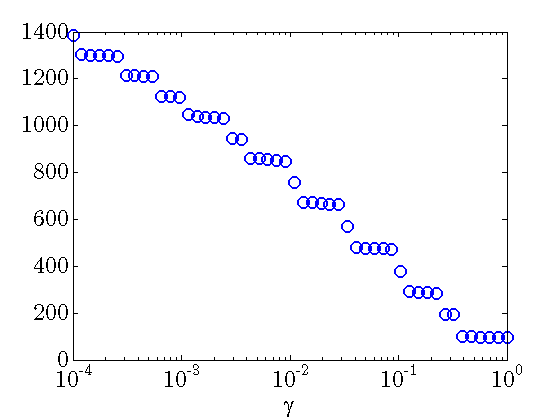The number of nonzero elements in the feedback gainas a function of. For a system with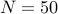masses, there are total of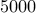elements in.

The number of nonzero sub-diagonals in both position and velocity feedback gains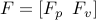decreases with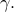For large values ofboth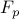and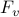become diagonal matrices.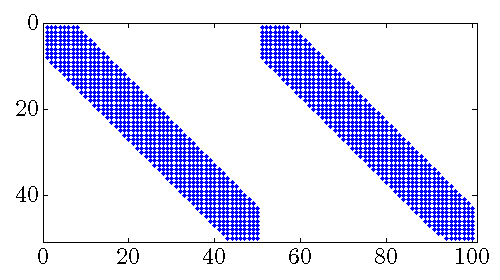Sparsity pattern of the feedback gain matrixfor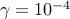.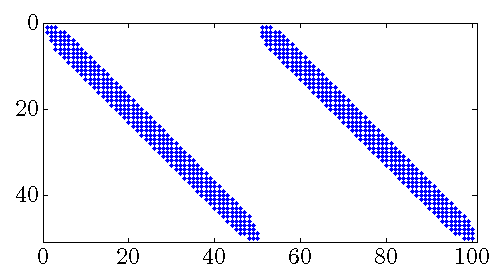Sparsity pattern of the feedback gain matrixfor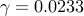.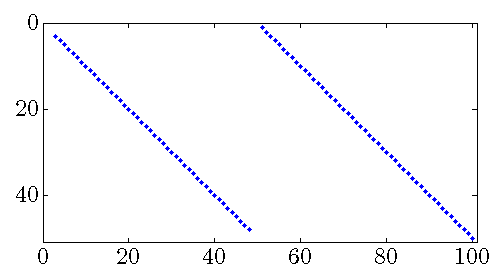Sparsity pattern of the feedback gain matrixfor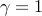.

### Performance of sparse feedback gains

In the absence of sparsity constraints, i.e., at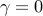, the optimal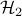controller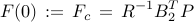is obtained from the positive definite solution of the algebraic Riccati equation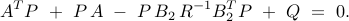Asincreases, the feedback gainbecomes sparser and the quadratic performance deteriorates.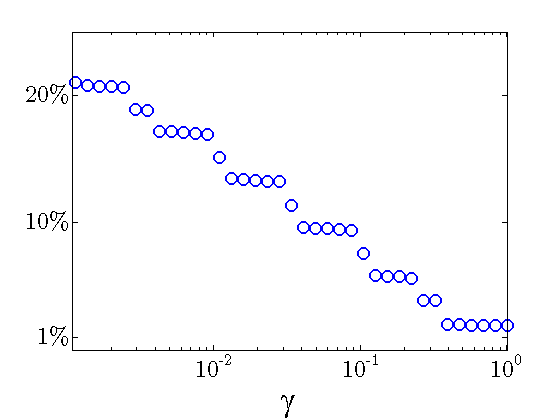Sparsity level: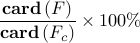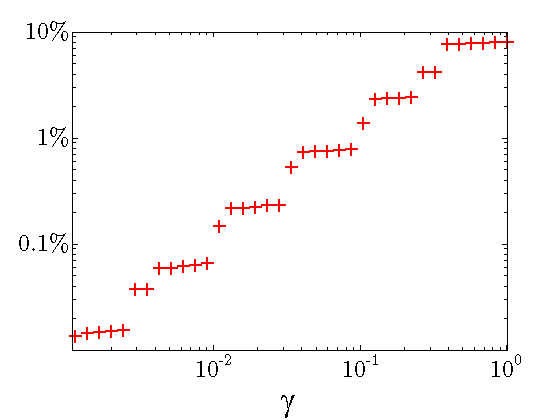Performance loss: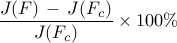The above results demonstrate that the optimal sparse feedback gain, with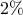of nonzero elements relative to the centralized feedback gain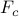, introduces performance loss of only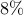compared to.

Sparsity vs. performance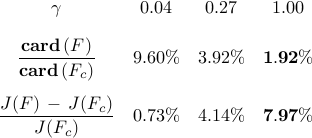### Diagonals of sparse feedback gains

The elements on the main diagonals of the optimal sparse feedback gains become larger as the number of the sub-diagonals ofdrops. Thus, in order to compensate for communication with smaller number of neighboring masses, each mass places more emphasis on its own position and velocity when forming control action.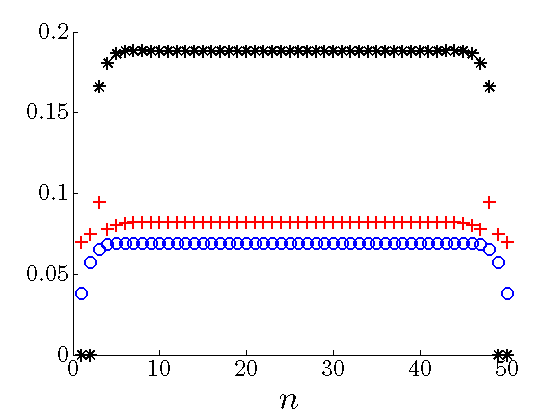Diagonals of the optimal sparsefor different values of: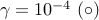,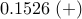,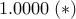. Blue circles are almost on the top of the main diagonal of the optimal centralized.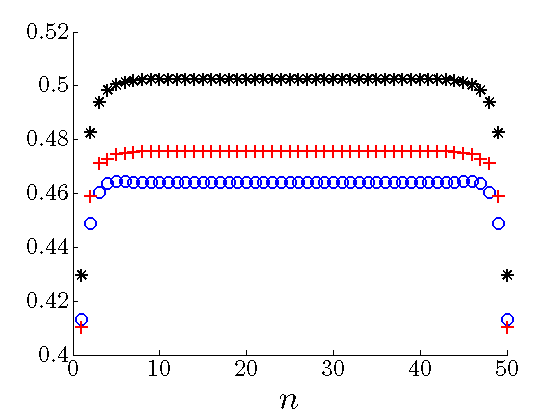Diagonals of the optimal sparsefor different values of:,,. Blue circles are almost on the top of the main diagonal of the optimal centralized.

Also see computational results obtained using other sparsity-promoting functions.

Back to mass-spring system example